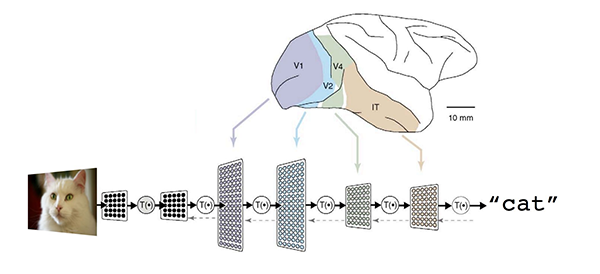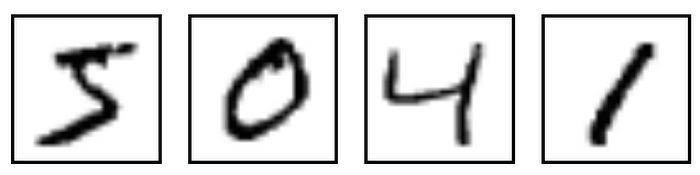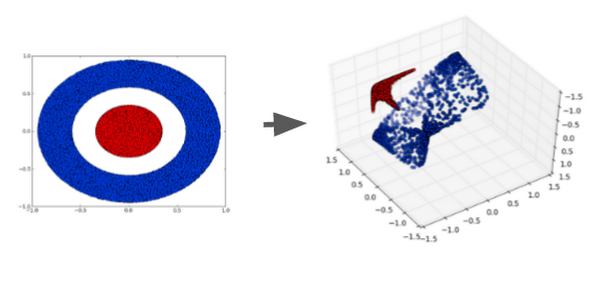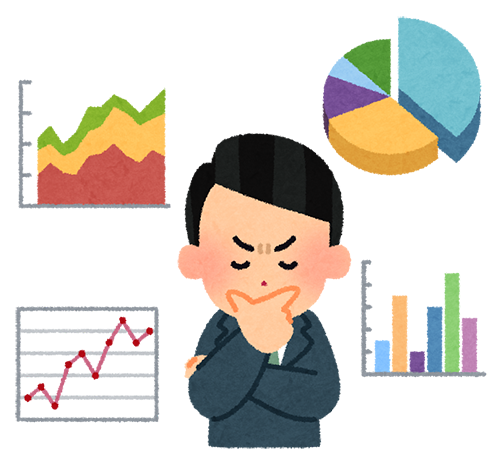AI & Machine Learning

# Understanding neural networks with TensorFlow Playground

You may have heard the buzz about neural networks and deep learning, and want to learn more. But when you learn about the technology from a textbook, many people find themselves overwhelmed by mathematical models and formulas. I certainly was.

For people like me, there's an awesome tool to help you grasp the idea of neural networks without any hard math: TensorFlow Playground, a web app written in JavaScript that lets you play with a real neural network running in your browser and click buttons and tweak parameters to see how it works.

In this article, I'd like to show how you can play with TensorFlow Playground so that you can understand the core ideas behind neural networks. Then you can understand why people have become so excited by the technology as of late.

### Let the computer solve the problem

Computer programming requires a programmer. Humans instruct a computer to solve a problem by specifying each and every step through many lines of code. But with machine learning and neural networks, you can let the computer try to solve the problem itself. A neural network is a function that learns the expected output for a given input from training datasets.A neural network is a function that learns from training datasets (From: Large-Scale Deep Learning for Intelligent Computer Systems, Jeff Dean, WSDM 2016, adapted from Untangling invariant object recognition, J DiCarlo et D Cox, 2007)

For example, to build a neural network that recognizes images of a cat, you train the network with a lot of sample cat images. The resulting network works as a function that takes a cat image as input and outputs the "cat" label. Or — to take a more practical example — you can train it to input a bunch of user activity logs from gaming servers and output which users have a high probability of conversion.

How does this work? Let's look at a simple classification problem. Imagine you have a dataset such as the one below. Each data point has two values: x1 (the horizontal axis) and x2 (the vertical axis). There are two groups of data points, the orange group and blue group.

How do you write code that classifies whether a data point is orange or blue? Perhaps you draw an arbitrary diagonal line between the two groups like below and define a threshold to determine in which group each data point belongs.

The condition of your IF statement would look like this.

where b is the threshold that determines the position of the line. By putting w1 and w2 as weights on x1 and x2 respectively, you make your code more reusable.

Further, if you tweak the values of w1 and w2, you can rotate the angle of the line as you like. You can also tweak the "b" value to move the line position. So you can reuse this condition for classifying any datasets that can be classified by a single straight line.

But the thing is, the programmer has to find appropriate values for w1, w2 and b — the so-called parameters — and instruct the computer how to classify the data points.

### A neuron: classifying a data point into two kinds

Now, let's look at how the computer behind TensorFlow Playground solves this particular problem. On the Playground, click the Play button in the upper left corner. The line between blue and orange data points begins to move slowly. Hit the reset button and click play again a few times to see how the line moves with different initial values. What you're seeing is the computer trying to find the best combination of weights and threshold to draw the straight line between two groups.

TensorFlow Playground is using a single artificial neuron for this classification. What's an artificial neuron? It’s an idea inspired by the behavior of biological neurons in the human brain.

For a detailed description about the mechanism of a biological neural network, visit the Wikipedia page: each neuron gets excited (activated) when it receives electrical signals from other connected neurons. Each connection between neurons has different strengths. Some connections are strong enough to activate other neurons whereas some connections suppress activation. Together, the hundreds of billions of neurons and connections in our brain embody human intelligence.

The research into biological neurons led to the creation of a new computing paradigm, the artificial neural network. With artificial neural networks, we mimic the behavior of biological neurons with simple mathematics. To solve the above classification problem, you can use the following simple neural network, which features a single neuron (aka Perceptron).

x1 and x2 are the input values, and w1 and w2 are weights that represent the strength of each connection to the neuron. b is the so-called bias, representing the threshold to determine whether or not a neuron is activated by the inputs. This single neuron can be calculated with the following formula.

Yes, that's exactly the same formula we used for classifying the datasets with a straight line. And actually, that's the only thing an artificial neuron can do: classify a data point into one of two kinds by examining input values with weights and bias. With two inputs, a neuron can classify the data points in two-dimensional space into two kinds with a straight line. If you have three inputs, a neuron can classify data points in three-dimensional space into two parts with a flat plane, and so on. This is called "dividing n-dimensional space with a hyperplane."

A neuron classifies any data point into one of two kinds

### Using a single neuron to perform image recognition

How can a "hyperplane" solve everyday problems? As an example, imagine you have lots of handwritten text images like below.Pixel images of handwritten texts (From: MNIST For ML Beginners, tensorflow.org)

You can a train single neuron to classify a set of images as "images of number 8" or "other images."

How do you do that? At first, you need to prepare tens of thousands of sample images for training. Let’s say a single image has 28 x 28 grayscale pixels; it will fit to an array with 28 x 28 = 784 numbers. Given 55,000 sample images, you'd have an array with 784 x 55000 numbers.

For each sample image in the 55K samples, you input the 784 numbers into a single neuron, along with the training label as to whether or not the image represents an "8."

As you saw on the Playground demo, the computer tries to find an optimal set of weights and bias to classify each image as an "8" or not.

After training with the 55K samples, this neuron will have generated a set of weights such as the ones below, where blue represents a positive value and red is a negative value.

That's it. Even with this very primitive single neuron, you can achieve 90% accuracy when recognizing a handwritten text image1. To recognize all the digits from 0 to 9, you would need just ten neurons to recognize them with 92% accuracy.

Again, the only thing this neuron can do is classify a data point as one of two kinds: "8" or not. What qualifies as a “data point" here? In this case, each image contains 28 x 28 = 784 numbers. In mathematical parlance, you could say that each image represents a single point in 784-dimensional space. The neuron divides the 784-dimensional space into two parts with a single hyperplane, and classifies each data point (or image) as "8" or not. (Yes, it's almost impossible to imagine what that dimensional space and hyperplane might look like. Forget about it.)

In the example above, we used handwritten text image1 as our sample data, but you can use a neural network to classify many kinds of data. For example, an online game provider could identify players that are cheating by examining player activity logs. An e-commerce provider can identify premium customers from web server access logs and transaction histories. In other words, you can express any data that can be converted and expressed as a number as a data point in n-dimensional space, let the neuron try to find the hyperplane, and see whether it helps you effectively classify your problem.

### How do you train a neural network?

As you can see a neural network is a simple mechanism that’s implemented with basic math. The only difference between the traditional programming and neural network is, again, that you let the computer determine the parameters (weights and bias) by learning from training datasets. In other words, the trained weight pattern in our example wasn’t programmed by humans.

In this article, I won’t discuss in detail how you can train the parameters with algorithms such as backpropagation and gradient descent. Suffice it to say that the computer tries to increase or decrease each parameter a little bit to see how it reduces the error compared with training dataset, in hopes of finding the optimal combination of parameters.

Think of the computer as a student or junior worker. In the beginning, the computer makes many mistakes and it takes some time before it finds a practical way of solving real-world problems (including possible future problems) and minimizes the errors (so called generalization).A neural network needs training time before it can minimize errors (From: Irasutoya.com)

We may revisit the topic in a future article. For now, content yourself with the fact that a neural network library such as TensorFlow encapsulates most of the necessary math for training, and you don’t have to worry too much about it.

### More neurons, more features to extract

We’ve demonstrated how a single neuron can perform a simple classification, but you may be wondering how a simple neuron can be used to build a network that can recognize thousands of different images and compete with a professional Go player? There's a reason why neural networks can get much smarter than what we described above. Let's take a look at another example from TensorFlow Playground.

This dataset can not be classified by a single neuron, as the two groups of data points can't be divided by a single line. This is a so-called nonlinear classification problem. In the real world, there's no end to non-linear and complex datasets such as this one, and the question is how to capture these sorts of complex patterns?

The answer is to add a hidden layer between the input values and output neuron. Click here to try it out.

What's happening here? If you click each one of the neurons in the hidden layer, you see they're each doing a simple, single-line classification:

• The first neuron checks if a data point is on the left or right
• The second neuron checks if it's in the top right
• The third one checks if it's in the bottom right
These three results are called features of the data. Outputs from these neurons indicate the strength of their corresponding features.

Finally, the neuron on the output layer uses these features to classify the data. If you draw a three dimensional space consisting of the feature values, the final neuron can simply divide this space with a flat plane. This is an example of a transformation of the original data into a feature space.

For some great visual examples of transformations, visit colah's blog.A hidden layer transforms inputs to feature space, making it linearly classifiable (From: Visualizing Representations: Deep Learning and Human Beings and Neural Networks, Manifolds and Topology, Christopher Olah)

In the case of the Playground demo, the transformation results in a composition of multiple features corresponding to a triangular or rectangular area. If you add more neurons by clicking the "plus" button, you'll see that the output neuron can capture much more sophisticated polygonal shapes from the dataset.

Getting back to the office worker analogy, you can say the transformation is extracting the insights that an experienced professional has in their daily work. A new employee gets confused and distracted by random signals coming from e-mails, phones, the boss, customers, etc., but senior employees are very efficient about extracting the essential signal from those inputs, and organize the chaos according to a few important principles.

Neural networks work the same way — trying to extract the most important features in a dataset to solve the problem. That's why neural networks can sometimes get smart enough to handle some pretty complex tasks.Neural network can extract insights from (seemingly) random signals (From: Irasutoya.com)

### We need to go deeper: building a hierarchy of abstractions

With more neurons in a single hidden layer, you can capture more features. And having more hidden layers means more complex constructs that you can extract from the dataset. You can see how powerful this can be in the next example.

What kind of code would you write to classify this dataset? Dozens of IF statements with many many conditions and thresholds, each checking which small area a given data point is in? I personally wouldn’t want to do that.

This is where machine learning and neural networks exceed the performance of a human programmer. Click here to see it in action (it will take a couple of minutes to train).

Pretty cool, isn't it? What you just saw was the computer trying to build a hierarchy of abstraction with a deep neural network. The neurons in the first hidden layers are doing the same simple classifications, whereas the neurons in the second and third layers are composing complex features out of the simple features, eventually coming up with the double spiral pattern.

More neurons + a deeper network = more sophisticated abstraction. This is how simple neurons get smarter and perform so well for certain problems such as image recognition and playing Go.

Some published examples of visualization by deep networks show how they're trained to build the hierarchy of recognized patterns, from simple edges and blobs to object parts and classes.

### Two challenges: computational power and training data

In this article, we looked at some TensorFlow Playground demos and how they explain the mechanism and power of neural networks. As you've seen, the basics of the technology are pretty simple. Each neuron just classifies a data point into one of two kinds. And yet, by having more neurons and deep layers, a neural network can extract hidden insights and complex patterns from a training dataset and build a hierarchy of abstraction.

The question is then, why isn't everybody using this great technology yet? There are two big challenges for neural networks right now. The first is that training deep neural networks requires a lot of computation power, and the second is that they require large training data sets. It can take several days or even weeks for a powerful GPU server to train a deep network with a dataset of millions of images.

Also, it takes a lot of trial and error to get the best training results with many combinations of different network designs and algorithms. Today, some researchers use tens of GPU servers or even supercomputers to perform large-scale distributed training.

But in very near future, fully managed distributed training and prediction services such as Google Cloud Machine Learning with TensorFlow may solve these problems with the availability of cloud-based CPUs and GPUs at an affordable cost, and may open the power of large and deep neural networks to everyone.

### Acknowledgement

Thanks so much David Ha, Etsuji Nakai, Christopher Olah and Alexandra Barrett for reviewing and giving such valuable comments on the post as well as refining the text. And special thanks to the authors of TensorFlow Playground, Daniel Smilkov, Shan Carter and D. Sculley, for the truly awesome work.

https://www.tensorflow.org/versions/r0.9/tutorials/mnist/beginners/index.html